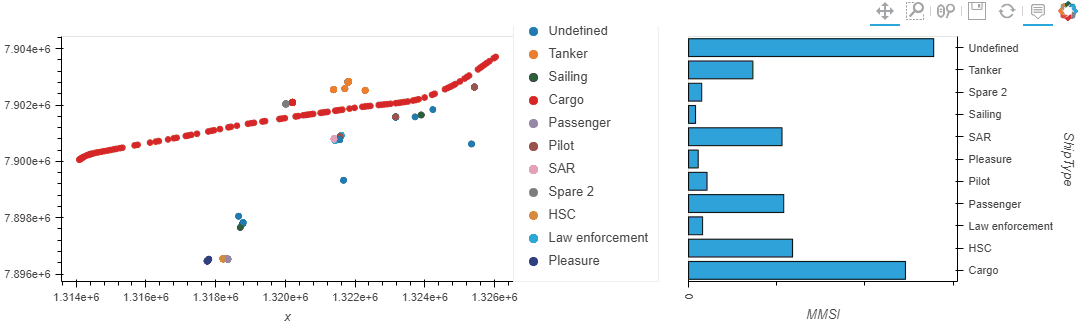# Linked brushing with bar chart

What is the easiest way to link a scatter plot and a bar chart?

Linked brushing does not support bar charts (Linked Brushing — HoloViews v1.17.1) but https://awesome-panel.org/kickstarter_dashboard shows that it is still possible to link a scatter plot and a bar chart (i.e. when we zoom into the scatter plot, the bar plot is filtered) but the code is far from a minimum working example and – since it is dated 2019 – I’m wondering if there are newer, more straightforward approaches?

Here’s the minimum example of scatterplot + bar, I’d like to start with: https://github.com/movingpandas/movingpandas-examples/blob/main/3-tech-demos/linked-brushing.ipynb (cell no. 7):

Ok, found it thanks to Linked tap selections in stacked bar plots - #2 by Marc and RangeXY — HoloViews v1.17.1

``````def selected_bar_plot(x_range, y_range):
if x_range:
tmp = df[(df.x>x_range)&(df.x<x_range)]
else:
tmp = df
return tmp.groupby("ShipType").count().hvplot.bar(x="ShipType", y='MMSI', width=400, rot=90)

map_plot = df.hvplot.scatter(x='x', y='y', c="ShipType", width=700, height=300)
rangexy = hv.streams.RangeXY(source=map_plot)

map_plot << hv.DynamicMap(selected_bar_plot, streams=[rangexy])
``````2 Likes